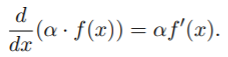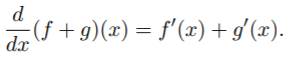# Linearity of Differentiation

Linearity of differentiation (also called the rule of linearity or the superposition rule for differentiation) is a combination of the sum rule and constant factor rule.

The property, which is essentially just an extension of the limit laws, states that differentiation respects linear combinations of functions. In other words, the act of differentiation is linear; If you differentiate a linear combination of functions, you’ll get the same linear combination of their derivatives.

## The Linearity of Differentiation Property

Linearity of differentiation is actually made up of two properties (Reinholz, 2020): The constant factor ruleand the sum ruleThese two properties, along with the power rule and the fact that the derivative of a constant is zero, leads to a few important observations, including:

## Why Do We Need This?

In elementary calculus, you’ll be working with the sum rule and constant factor rules individually. You probably won’t even realize that they are part of the same property, nor will you likely use the property. Where linearity of differentiation really comes into play is in higher math classes, when you’ll use the property (along with many others) to prove something else. For example:

• Peters (2014) uses the property as part of a proof showing that G1 constructions yield C1 iso-geometric elements.
• Sacks (1985) uses the same property for a completely different purpose: to discuss where a singularity might occur.

## References

Geyer, L. (2016). Stat 8931 (Exponential Families) Lecture Notes The Eggplant that Ate Chicago (the Notes that Got out of Hand). Retrieved September 2, 2020 from: http://www.stat.umn.edu/geyer/8931expfam/convex.pdf
Lee. J. Differentiation Rules I [Secs. 3.1 & 3.2]. Retrieved September 2, 2020 from: http://oregonstate.edu/instruct/mth251/Jwlee/251H_001_F2004/Sec3-1&3-2.pdf
Peters, J. (2014). Matched G1-constructions yield C1-continuous isogeometric elements. Retrieved September 2, 2020 from: http://citeseerx.ist.psu.edu/viewdoc/download?doi=10.1.1.746.3255&rep=rep1&type=pdf
Reinholz, D. Differentiation Rules. Retrieved September 2, 2020 from: https://www.ocf.berkeley.edu/~reinholz/ed/08fa_m160/lectures/differentiation_rules.pdf
Sacks, E. (1985). Qualitative mathematical reasoning. Retrieved September 2, 2020 from: http://groups.csail.mit.edu/medg/ftp/sacks/sacks85.tex

CITE THIS AS:
Stephanie Glen. "Linearity of Differentiation" From StatisticsHowTo.com: Elementary Statistics for the rest of us! https://www.statisticshowto.com/linearity-of-differentiation/